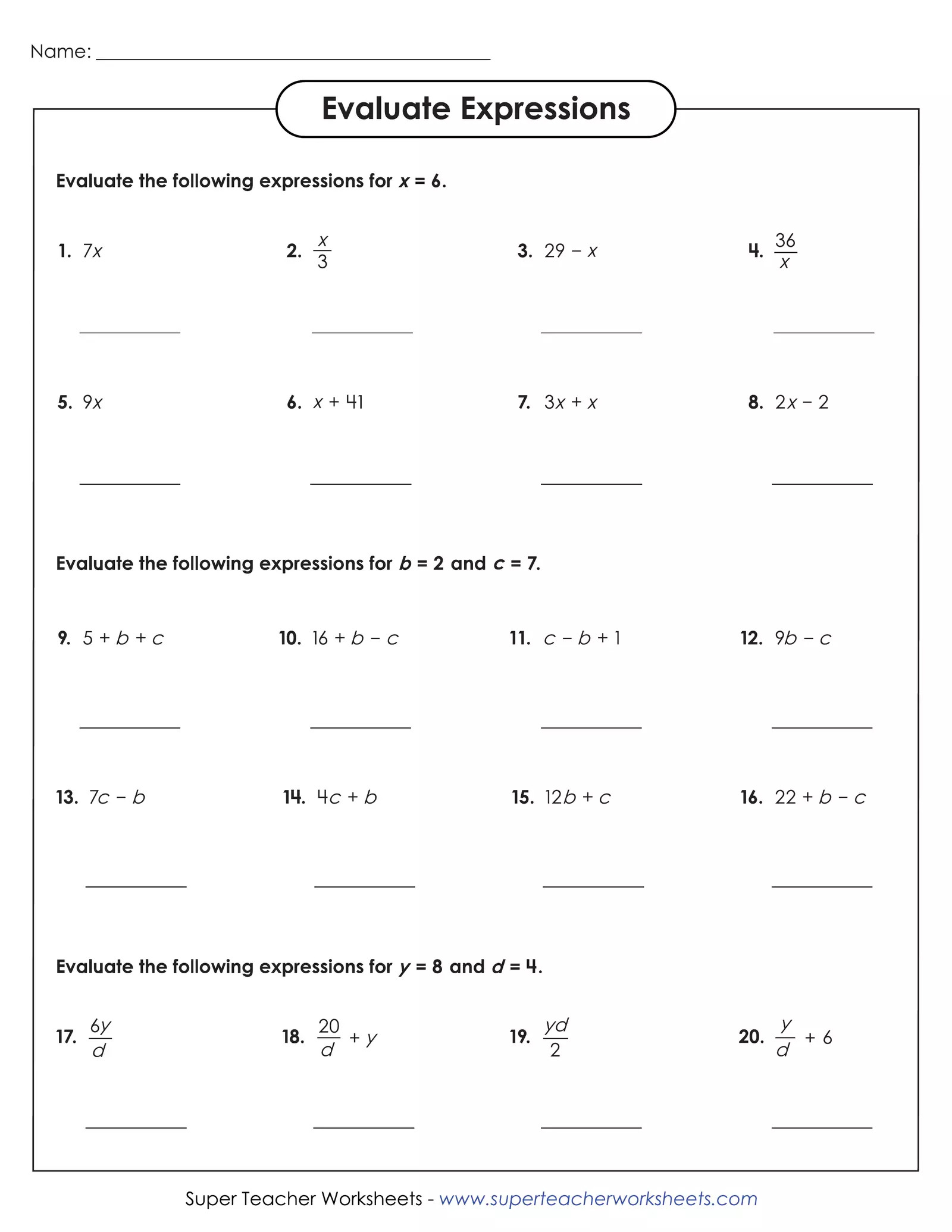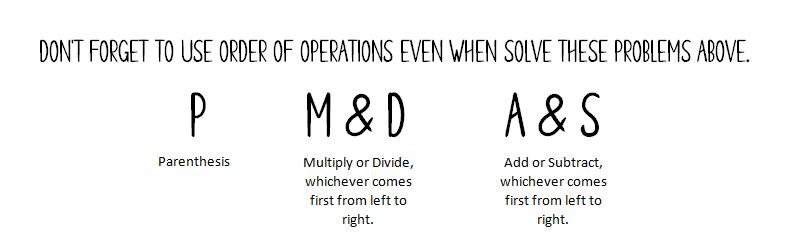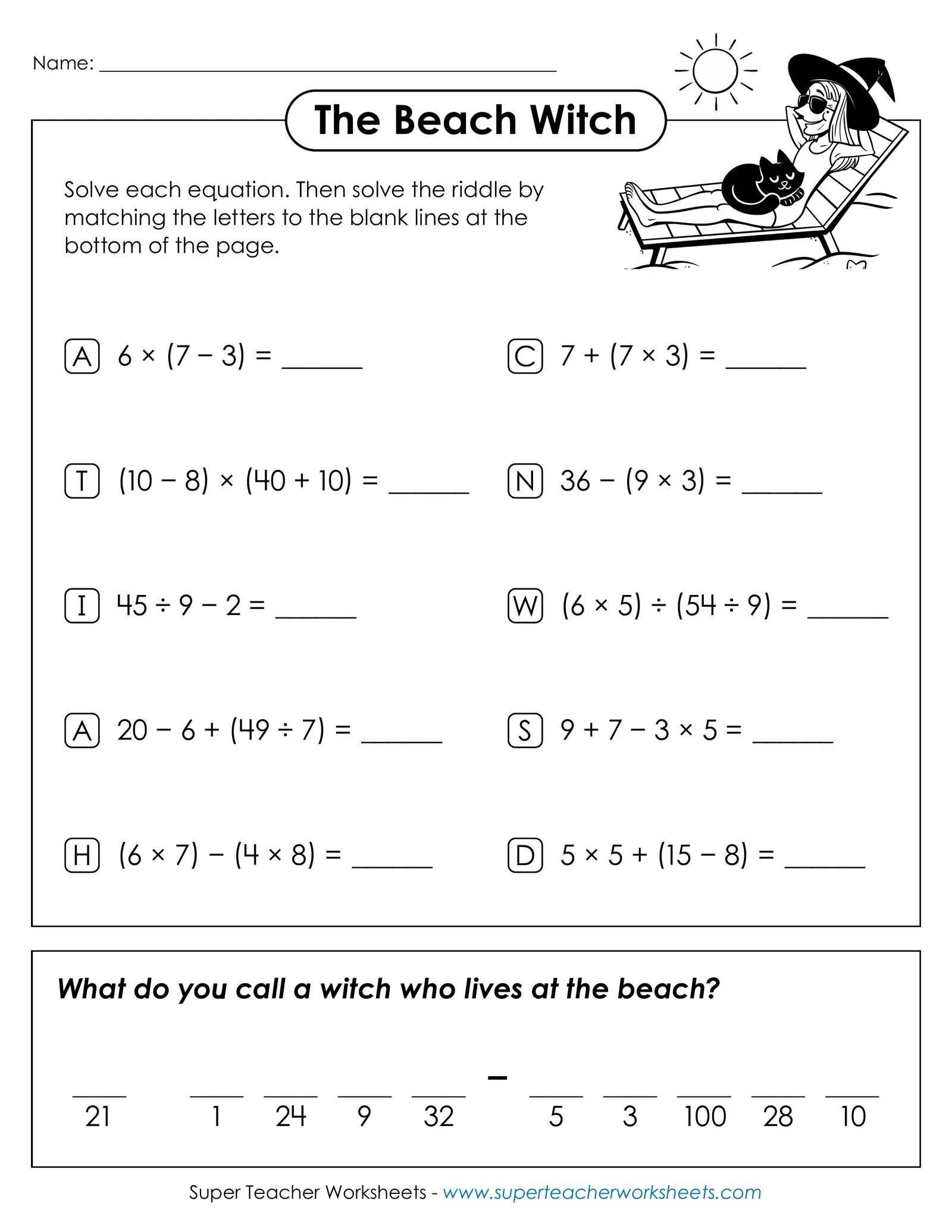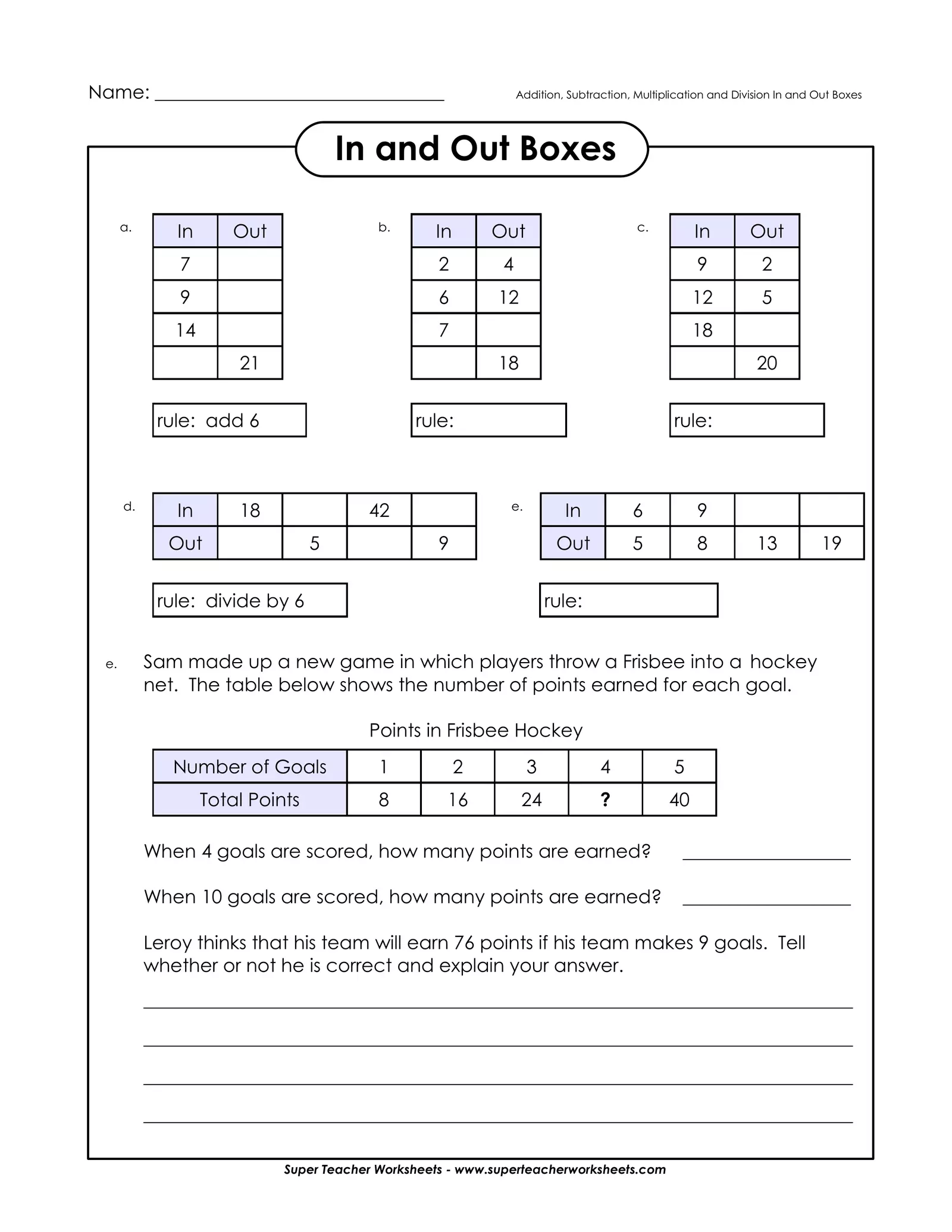Best for live in-class or video conferencing lessonsStart teacher-led lessonThis problem just means to divide.
This problem just means to divide.Name: _________________________________________________ The Beach Witch Solve each equation. Then solve the riddle by matching the letters to the blank lines at the bottom of the page. A 6 × (7 − 3) = ______ C 7 + (7 × 3) = ______ T (10 − 8) × (40 + 10) = ______ N 36 − (9 × 3) = ______ I 45 ÷ 9 − 2 = ______ W (6 × 5) ÷ (54 ÷ 9) = ______ A 20 − 6 + (49 ÷ 7) = ______ S 9 + 7 − 3 × 5 = ______ H (6 × 7) − (4 × 8) = ______ D 5 × 5 + (15 − 8) = ______ What do you call a witch who lives at the beach? _____ _____ _____ _____ _____ --- _____ _____ _____ _____ _____ 21 1 24 9 32 5 3 100 28 10 Super Teacher Worksheets - www.superteacherworksheets.comName: _______________________________ Addition, Subtraction, Multiplication and Division In and Out Boxes In and Out Boxes a. b. c. In Out In Out In Out 7 2 4 9 2 9 6 12 12 5 14 7 18 21 18 20 rule: add 6 rule: rule: d. e. In 18 42 In 6 9 Out 5 9 Out 5 8 13 19 rule: divide by 6 rule: e. Sam made up a new game in which players throw a Frisbee into a hockey net. The table below shows the number of points earned for each goal. Points in Frisbee Hockey Number of Goals 1 2 3 4 5 Total Points 8 16 24 ? 40 When 4 goals are scored, how many points are earned? __________________ When 10 goals are scored, how many points are earned? __________________ Leroy thinks that his team will earn 76 points if his team makes 9 goals. Tell whether or not he is correct and explain your answer. ____________________________________________________________________________ ____________________________________________________________________________ ____________________________________________________________________________ ____________________________________________________________________________ Super Teacher Worksheets - www.superteacherworksheets.com